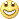# Requests & Ideas - page 403163390

rosalieone:
Is it calculating speed as first derivative of the indicator's data ? https://www.mathsisfun.com/calculus/derivatives-introduction.html

It does not

This is what it calculates :

V[pos]/V[pos+Acceleration_Length]

"Slope" from that page can not be calculated for a financial time series (x axis is time, y axes is price : they are incompatible)193

Sorry, you mean first derivative (speed) of the indicator is not possible ?182

Mladen, the attached volty channel stop on jurik summed histo unlimited 2.1 indicator is giving exactly the same values for price type 2 and price type 3 when i use only one parameter set in the indicator.

I double checked it with volty channel stop v2.2 mtf nmc indicator and the same thing happens there as well. (exactly same results when price type is 2 and price type is 3)

Is it due to the nature of the calculation of the indicator or is there a small glitch?

By the way, in the meantime can you please update the attached indicator with your latest price types list?163390

rosalieone:
Sorry, you mean first derivative (speed) of the indicator is not possible ?

rosalieone

You asked me what does the indicator you posted calculate. That indicator does not calculate what is shown on that page (not even close), and what I tried to explain that due to the incompatibility of time series x axis and y axis data, expression like :

Slope = Change in Y / Change in X

shown on that page from the link you provided, that formula is not usable (it does not produce usable results). Now, since that part (the first step) of calculation is not usable, and since the rest depends on that first step, that calculation from there will produce wrong results if applied to financial time series193

Ok understoodrosalieone

You asked me what does the indicator you posted calculate. That indicator does not calculate what is shown on that page (not even close), and what I tried to explain that due to the incompatibility of time series x axis and y axis data, expression like :

Slope = Change in Y / Change in X

shown on that page from the link you provided, that formula is not usable (it does not produce usable results). Now, since that part (the first step) of calculation is not usable, and since the rest depends on that first step, that calculation from there will produce wrong results if applied to financial time series182

Hi Mladen, Did you see my msg? Volty channel stop on jurik indicators give same results for price type 2 and 3. Is it due to the nature of the calcaluation of the indicator?

mandagozu81:
Mladen, the attached volty channel stop on jurik summed histo unlimited 2.1 indicator is giving exactly the same values for price type 2 and price type 3 when i use only one parameter set in the indicator.

I double checked it with volty channel stop v2.2 mtf nmc indicator and the same thing happens there as well. (exactly same results when price type is 2 and price type is 3)

Is it due to the nature of the calculation of the indicator or is there a small glitch?

By the way, in the meantime can you please update the attached indicator with your latest price types list?163390

mandagozu81:
Hi Mladen, Did you see my msg? Volty channel stop on jurik indicators give same results for price type 2 and 3. Is it dueto the nature of the calcaluation of the indicator?

mandagozu81

Check code lines 158 through 162 :

if(vchprices==2 || vchprices==3)

{

double bprice = iDSmooth(iMA(NULL,0,1,0,MODE_SMA,PRICE_HIGH,i),smlengths,smphases,(bool)smdoubles,i, 0);

double sprice = iDSmooth(iMA(NULL,0,1,0,MODE_SMA,PRICE_LOW, i),smlengths,smphases,(bool)smdoubles,i,20);

}

As you can see for prices 2 or 3 (high and low) the result will always be the same. That is the way how it is calculated142

Hi, how do you set the anchor at the beginning of the line. Should I drag and drop it on the chart or put a figure on the indicator ?

Thanks

Kiko

Files:163390

kiko12:
Hi, how do you set the anchor at the beginning of the line. Should I drag and drop it on the chart or put a figure on the indicator ?

Thanks

Kiko

Kiko

Set the anchor parameter to the bar you wish it to be anchored to, but the anchoring is to the bar number (not to the bar fixed in time)182

Thank you for the clarification Mladen.

mandagozu81

Check code lines 158 through 162 :

if(vchprices==2 || vchprices==3)

{

double bprice = iDSmooth(iMA(NULL,0,1,0,MODE_SMA,PRICE_HIGH,i),smlengths,smphases,(bool)smdoubles,i, 0);

double sprice = iDSmooth(iMA(NULL,0,1,0,MODE_SMA,PRICE_LOW, i),smlengths,smphases,(bool)smdoubles,i,20);

}

As you can see for prices 2 or 3 (high and low) the result will always be the same. That is the way how it is calculated